## The Markov Decision Process (MDP)

Learn about the Markov Chain and the Markov Decision Process in this guest post by Sudarshan Ravichandran, a data scientist and AI enthusiast, and the author of Hands-On Reinforcement Learning with Python.

A mathematical framework for solving reinforcement learning(RL) problems, the Markov Decision Process (MDP) is widely used to solve various optimization problems. MDP provides a mathematical framework for solving RL problems, andalmost all RL problems can be modeled as MDP. This tutorial will take you through the nuances of MDP and its applications.

Before going into MDP, you must first understand the Markov chain and Markov process, which form the foundation of MDP.

The Markov property states that the future depends only on the present and not on the past. The Markov chain is a probabilistic model that solely depends on the current state to predict the next state and not the previous states.This means that the future is conditionally independent of the past. The Markov chain strictly follows the Markov property.

For example, if you know that the current state is cloudy, you can predict that the next state could be rainy. You came to the conclusion that the next state could be rainy only by considering the current state (cloudy) and not the past states, which might be sunny or windy.

However, the Markov property does not hold true for all processes. For example, throwing a dice (the next state) has no dependency on the previous number (the current state), whatsoever.

Moving from one state to another is called transition and its probability is called a transition probability. The transition probabilities can be formulated in the form of a table, as shown next, and it is called a Markov table. It shows, given the current state, what the probability of moving to the next state is:

 Current state Next state Transition probability Cloudy Rainy 0.6 Rainy Rainy 0.2 Sunny Cloudy 0.1 Rainy Sunny 0.1

You can also represent the Markov chain in the form a state diagram that shows the transition probability: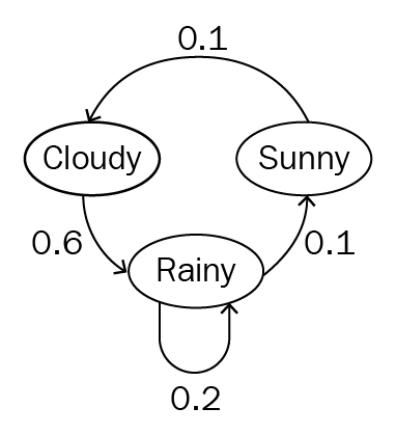The preceding state diagram shows the probability of moving from one state to another. Still don't understand the Markov chain? Okay, let’s talk.

Me: "What are you doing?"

You: "I'm reading about the Markov chain."

Me: "What is your plan after reading?"

You: "I'm going to sleep."

Me: "Are you sure you're going to sleep?"

You: "Probably. I'll watch TV if I'm not sleepy."

Me: "Cool; this is also a Markov chain."

You: "Eh?"

The above conversation can be formulated into a Markov chain. The state diagram will be as follows: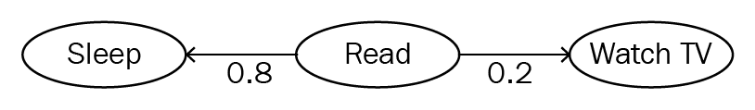The Markov chain lies in the core concept that the future depends only on the present and not on the past. A stochastic process is called a Markov process if it follows the Markov property.

MDP is an extension of the Markov chain,which provides a mathematical framework for modeling decision-making situations. MDP is represented by five important elements:

• A set of states the agent can actually be in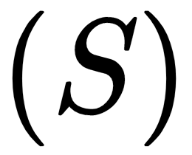A set of actions that can be performed by an agent, for moving from one state to another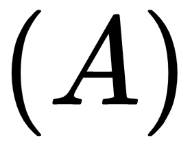• A transition probability,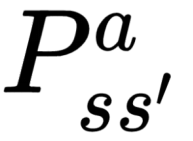, which is the probability of moving from one stateto another state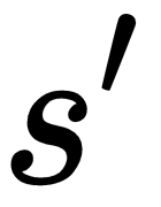by performing some action.• A reward probability,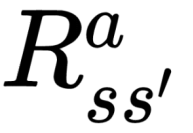, which is the probability of a reward acquired by the agent for moving from one stateto another state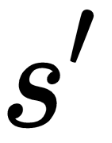by performing some action• A discount factor,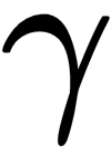, which controls the importance of immediate and future rewards. This is discussed in detail in the next section.

### Rewards and returns

In an RL environment, an agent interacts with the environment by performing an action and moves from one state to another. Based on the action it performs, it receives a reward. A reward is nothing but a numerical value, say, +1 for a good action and -1 for a bad action.

How do you decide if an action is good or bad? In a maze game, a good action is when the agent makes a move such that it doesn't hit a maze wall; a bad action is when the agent moves and hits the maze wall.

An agent tries to maximize the total amount of rewards (cumulative rewards) it receives from the environment instead of immediate rewards. The total amount of rewards the agent receives from the environment is called returns. So, you can formulate total amount of rewards (returns) received by the agents as follows: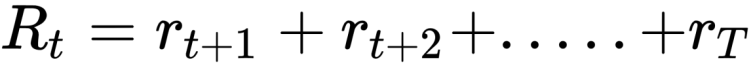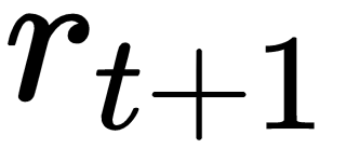is the reward received by the agent at a time step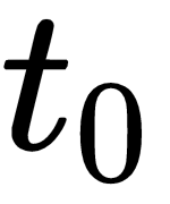while performing an action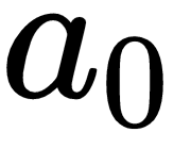to move from one state to another.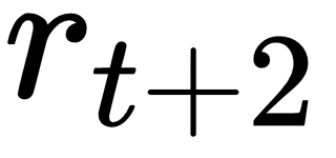is the reward received by the agent at a time step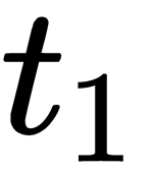while performing an action to move from one state to another. Similarly,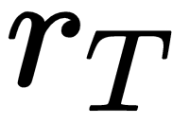is the reward received by the agent at a final time step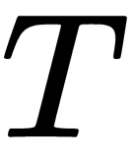while performing an action to move from one state to another.

### Episodic and continuous tasks

Episodic tasks are the tasks that have a terminal state (end). In RL, episodes are considered agent-environment interactions from initial to final states.

For example, in a car racing video game, you start the game (initial state) and play the game until it is over (final state). This is called an episode. Once the game is over, you start the next episode by restarting the game, and you will begin from the initial state irrespective of the position you were in the previous game. So, each episode is independent of the other.

In a continuous task, there is no terminal state. Continuous tasks will never end. For example, a personal assistance robot does not have a terminal state.

### Discount factor

You have seen that an agent’s goal is to maximize the return. For an episodic task, you can define the return as Rt= rt+1 + rt+2 + ..... +rT, where T is the final state of the episode.

Since there’s final state for a continuous task, you can define the return for continuous tasks as Rt= rt+1 + rt+2+....,which sums up to infinity. But how do you maximize the return if it never stops?

That's why the notion of a discount factor is introduced. You can redefine the return with a discount factor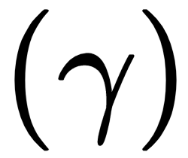, as follows: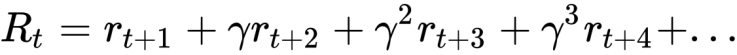... (1)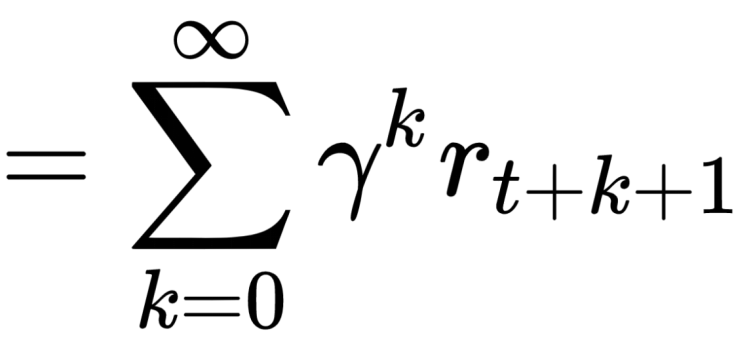… (2)

The discount factor decides how much importance can be given to the future rewards and immediate rewards. The value of the discount factor lies within 0 to 1. A discount factor of 0 means that immediate rewards are more important, while a discount factor of 1 would mean that future rewards are more important than immediate rewards.

A discount factor of 0 will never learn, considering only the immediate rewards; similarly, a discount factor of 1 will learn forever looking for a future reward, which may lead to infinity. So the optimal value of the discount factor lies between 0.2 and 0.8.

You give importance to immediate and future rewards depending on the use case. In some cases, future rewards are more desirable than immediate rewards and vice versa. In a chess game, the goal is to defeat the opponent's king.

If you give importance to the immediate reward, which is acquired by actions like pawn defeating any opponent player and so on, the agent will learn to perform this sub-goal instead of learning to reach the actual goal. In such a case, you must give importance to future rewards.

In some other cases, immediate rewards are preferred over future rewards. (Say, would you prefer chocolates today or 13 months later?)

### The policy function

The policy function can be represented as follows: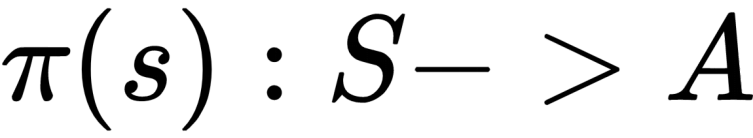This indicates mapping from states to actions. So, basically, a policy function tells the action to be performed in each state. The ultimate goal lies in finding the optimal policy that specifies the correct action to be performed in each state, which maximizes the reward.

### State value function

A state value function is also simply called a value function. It specifies how good it is for an agent to be in a particular state with a policy π. A value function is often denoted by V(s). It denotes the value of a state following a policy.

The state value function can be defined as follows: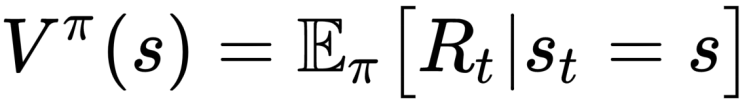This specifies the expected return starting from state, s according to policy, π. You can substitute the value of Rt in the value function from (2) as follows: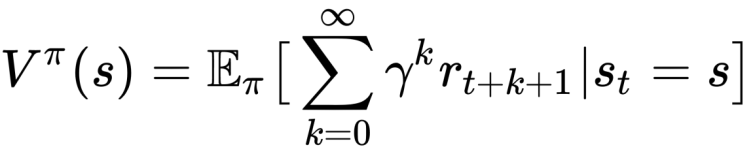Note that the state value function varies depending on the policy chosen.

You can view value functions in a table. Supposeyou have two states and both of these states follow the policy π. Based on the value of these two states, you can tell how good it is for the agent to be in that state following a policy. The greater the value, the better the state is:

 State Value State 1 0.3 State 2 0.9

Based on the above table, you can tell that it is good to be in state 2, as it has high value.

### State-action value function (Q function)

A state-action value function is also called the Q function. It specifies how good it is for an agent to perform a particular action in a state with a policy, π. The Qfunction is denoted by Q(s). It denotes the value of taking an action in a state following a policy π

The Q function is defined as follows: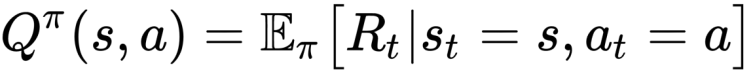This specifies the expected return starting from state, s with the action a according to policy π. You can substitute the value of Rt in the Q function from (2) as follows: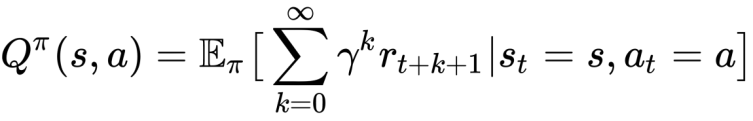The difference between the value function and the Q function is that the value function specifies the goodness of a state, while a Q function specifies the goodness of an action in a state.

Like state value functions, Q functions can also be viewed in a table. It is also called a Q table. Supposeyou have two states and two actions; the Q table looks as follows:

 State Action Value State 1 Action 1 0.5 State 1 Action 2 0.02 State 2 Action 1 0.03 State 2 Action 2 0.9

Thus, the Q table shows the value of all possible state action pairs. So, by looking at this table, you can come to the conclusion that performing action 1 in state 1 and action 2 in state 2 is the better option as it has high value.

This ends the discussion on MDP. To learn more about reinforcement learning, you can explore Hands-On Reinforcement Learning with Python. The book features an easy-to-follow style, taking you through everything from scratch with the support of rich examples written in Python. The book is a must-read for ML developers and deep learning enthusiasts who want to learn about reinforcement learning from scratch.

© copyright 2017 www.aimlmarketplace.com. All Rights Reserved.

A Product of HunterTech Ventures